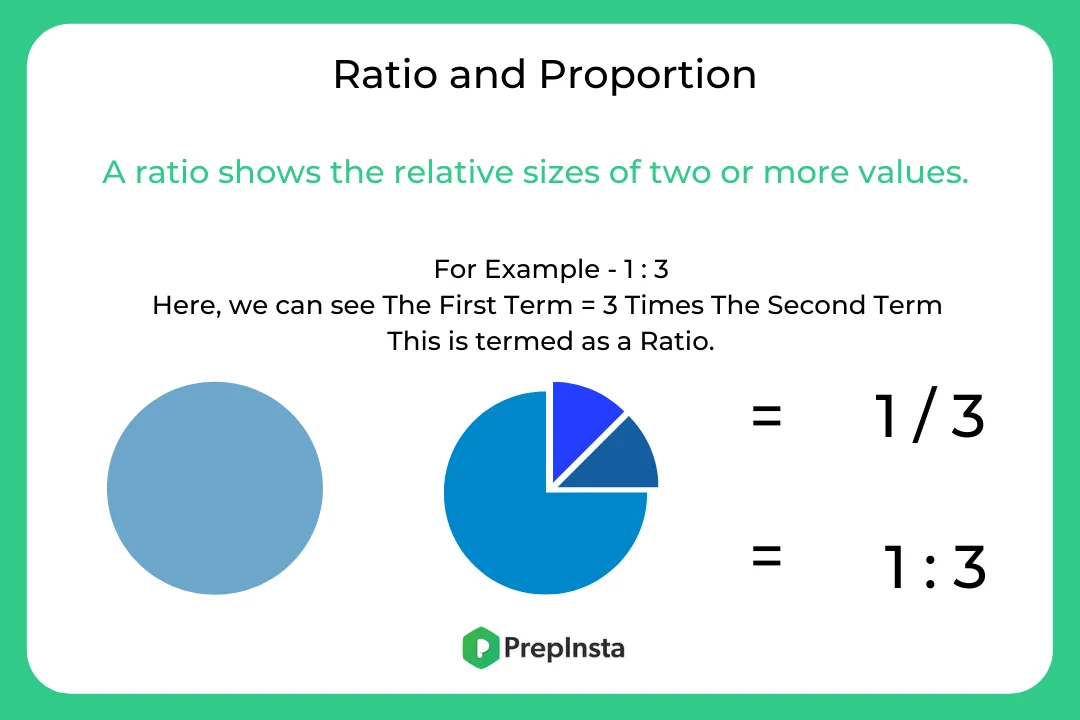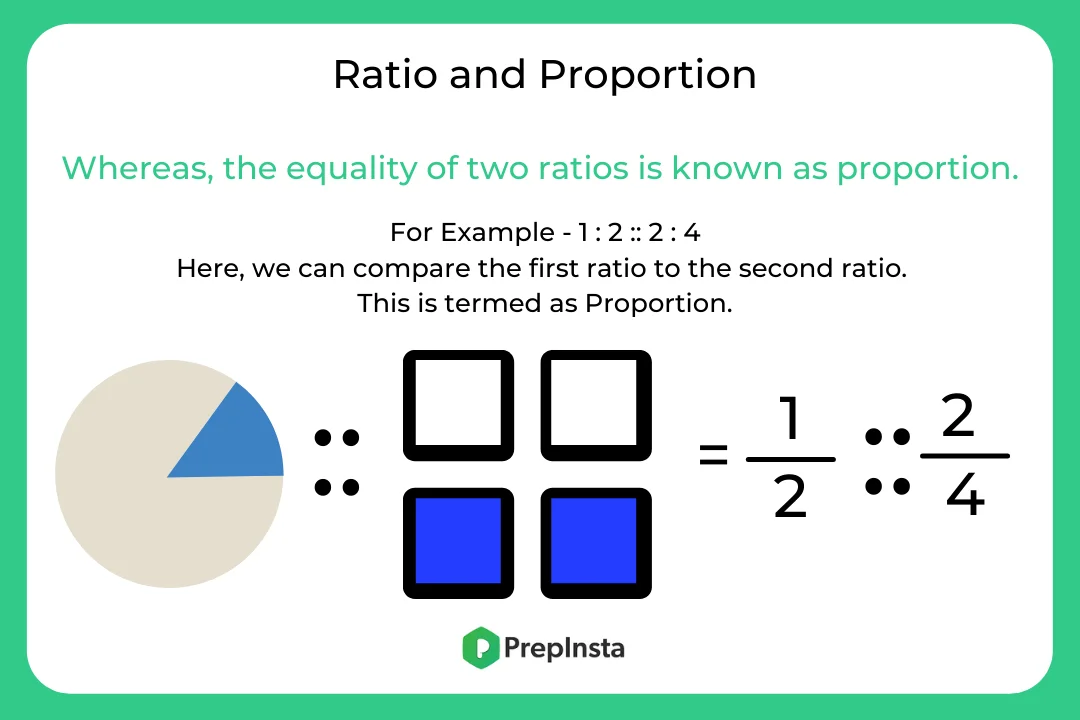# Formulas for Ratio and Proportions

## Basic Formulas of Ratio and Proportion

On this page we have discussed Ratio and Proportion formulas, definition with examples.

Ratio and Proportion are explained majorly based on fractions. When a fraction is represented in the form of a:b, then it is a ratio whereas a proportion states that two ratios are equal. Here, a and b are any two integers.### Proportion: –

The equality of two ratios is known as proportion. It is used to find out the quantity of one class over the total. In other words, the proportion is a part that describes the comparative relation with the overall part.

Subsequently, 2 : 3 equals to 4 : 6, we will write 2 : 3 :: 4 : 6 and we can say that 2, 3, 4, and 6 are in proportion. Consequently, 2, 3, 4, and 6 are called 1st, 2nd, 3rd, and 4th proportional respectively. The first and the fourth proportional are called the extreme terms while the second and the third proportional are called mean terms.

The product of the means equals the product of the extremes.### Related Banners

Get PrepInsta Prime & get Access to all 200+ courses offered by PrepInsta in One Subscription

### Formulas for Ratio and Proportions are as follows: –

 Fourth Proportional If x : y = z: a, then a is called the fourth proportional to x, y, z. Third Proportional If x: y = y: z, then z is called the third proportional to x and y. Mean Proportional Mean proportional between x and y is √xy Comparison of Ratios We say that (x: y) > (z: a), then (x/y) > (z /a). Compounded Ratio The compounded ratio of the ratios (x: y), (z: a), (b : c) is (xzb: yac)

### Formulas & Properties of Ratio

• The ratio of two people a and b is denoted as a : b.
• a : b = ma : mb, where m is a constant.
• x : y : z = X : Y : Z is equivalent to x/X=y/Y=z/Z
• If x/y = z/a  then, x+y/x-y  = z+a/z-a

### Property of Proportion

• x/y=z/a , this means x : y ::z:a

Question 1 : Sales manager of pepperfry.com was checking the inventory and concluded that the cost price of 2 tables and 3 chairs is Rs. 2200 and the cost price of 2 chairs and 4 tables is Rs. 2400. The question is what will be the ratio between the cost price of the chairs and the table ?

Options :

1. 8 : 9
2. 6 : 5
3. 9 : 5
4. 10 : 7

Solution : Let the cost price of one table = x Rs., the cost price of one chairs = y Rs.
According to the question,
2x + 3y = 2200 ….1
2y + 4x = 2400
2x + y = 1200 ….2
After solving these 2 equation,
x = 350 Rs. , y = 500 Rs.
Ratio = 500 : 350 = 10 : 7

Question 2 : When Kate and Jennifer received their income statement last week, their accountant noted that their income for 2017 was divided by five to four. Kate’s income in 2018 compared to that of 2017 is 3:5, while Jennifer’s income in 2018 compared to that of 2017 is 3:2. Find Jennifer’s salary for the year 2017 if the combined income of Kate and Jennifer in 2018 was Rs. 10242.

Options :

1. 2450 Rs
2. 4552 Rs
3. 1150 Rs
4. 4598 Rs

Solution : Let the income of Kate in 2018 and 2017 be 3x and 5x respectively.
Let the income of Jennifer in 2018 and 2017 be 3y and 2y respectively
Since, the ratio of their income in the year 2017 was 5 : 4
5x : 2y = 5 : 4
2x = y
The sum of their incomes in 2018 is Rs. 10242
3x + 3y = 10, 242
9x = 10, 242
x = 1,138 and y = 2276
Jennifer’s income for the year 2017 = 2y = Rs. 4552

Question 3 : Rick Grimes has 2 vessels A and B of equal volume containing Petrol and Diesel in the ratio 3 : 2 and 2 : 1 to their brim respectively. He then poured two litres of the solution from vessel A and three litres of the solution from vessel B into a big empty vessel C. If the solution in C occupied 40% of the capacity of C, what proportion would he achieve of the volume of vessel C which should be the volume of Diesel that shall be added so that the ratio of Petrol and Diesel in vessel C becomes 1 : 1?

Options :

1. 21 : 152
2. 22 : 120
3. 14 : 125
4. 14 : 150

Solution : Amount of Petrol poured into C from vessel A and B,
$=2\times \frac{3}{5}+3\times \frac{2}{3}=\frac{16}{5}litres$

Also, amount of Diesel poured into C from vessels A and B,
$=5-\frac{16}{5}=\frac{9}{5}litres$

Given, 5 litres rePresent 40% of the capacity of vessel C, vessel C has a capacity of,
$=5\times \frac{5}{2} = 12.5 litres$

To make the quantities of Diesel and Petrol same in the vessel C, quantity of Diesel to be added,
$=\frac{16}{5}-\frac{9}{5}= \frac{7}{5} litres$

Therefore, the required ratio is,
$=\frac{\frac{7}{5}}{12.5}= \frac{14}{125}litres$

Question 4: Vin Diesel distributed some chocolates among his four children and kept some with him. The eldest three children got chocolates in the ratio 3 : 11 : 7. The total number of chocolates with Vin and youngest child is three times the total chocolates with the three eldest children. The ratio of chocolates with Vin and that with all the children is 3 : 4. Find the total number of chocolates if the youngest child has 81 chocolates with him?
Options :

1. 252
2. 263
3. 274
4. 285

Solutions :
Let the children be P, Q, R and S and Mr Flynn be F

Chocolates with P : Q : R = 3 : 7 : 11

Let the number of chocolates be 3X, 7X and 11X

Total chocolates with three eldest children = 21X

Chocolate with F and S = 3 × 21X = 63X

Total chocolates = (21X + 63X) = 84X

Chocolate with F : (P + Q + R + S) = 3 : 4

Total 7 units of chocolate = 84 X

1 unit = 12X

Chocolate with F = 3 × 12X = 36X

Chocolate with S =  (63X – 36X) = 27X

27 X = 81  →  X=3

Total number of chocolates = 84X = 84 × 3 = 252

Question 5 : Tyris Gibson has scored the marks which are in the ratio of 7 : 10 in theory exams. Total marks which can be obtained in practicals were 20% of the total marks of theory. If Tyris got full marks in practical then find the ratio of total marks obtained by Tyris to the total marks which can be obtained in the subject.
Options :

1. 4 : 5
2. 3 : 5
3. 2 : 4
4. 3 : 4

Solution :
Let, marks obtained by Tyris Gibson in theory and total marks of theory be ‘7x’ and ‘10x’ respectively

So, total marks (theory + practical) = 10x + 2x = 12x

Marks obtained by Tyris Gibson (theory + practical) = 7x + 2x = 9 x

$Required Ratio = \frac{9x}{12x}=\frac{3}{4}$

## Get over 200+ course One Subscription

Courses like AI/ML, Cloud Computing, Ethical Hacking, C, C++, Java, Python, DSA (All Languages), Competitive Coding (All Languages), TCS, Infosys, Wipro, Amazon, DBMS, SQL and others